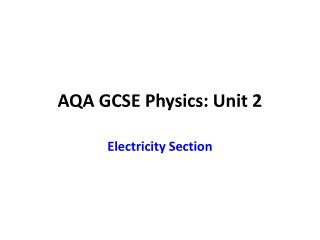DownloadDownload PresentationAQA GCSE Physics: Unit 2

# AQA GCSE Physics: Unit 2

Télécharger la présentation## AQA GCSE Physics: Unit 2

- - - - - - - - - - - - - - - - - - - - - - - - - - - E N D - - - - - - - - - - - - - - - - - - - - - - - - - - -
##### Presentation Transcript

1. AQA GCSE Physics: Unit 2 Electricity Section

2. Useful Information • AQA Specification can be found by clicking this link. http://store.aqa.org.uk/sciencelab/AQA-PHYS-W-SP.PDF • GCSE Bitesize relevant pages (Electricity section)http://www.bbc.co.uk/schools/gcsebitesize/science/add_aqa/electricity/ • S-cool physics current electricity section. http://www.s-cool.co.uk/gcse/physics/static-and-current-electricity

3. When certain insulating materials are rubbed against each other they become electrically charged. • Negatively charged electrons are rubbed off one material onto the other.

4. The material that gains electrons becomes negatively charged. • The material that loses electrons is left with an equal positive charge. • Have a look at these excellent simulations from the University of Colorado. • Balloons and Electricity  http://phet.colorado.edu/en/simulation/balloons • John Travoltage  http://phet.colorado.edu/en/simulation/travoltage

5. Attraction and Repulsion

6. Dangers of Static • Can you think of any dangers of static electricity?

7. Uses • Can you think of any uses of static electricity? • Some ideas here:  http://en.wikibooks.org/wiki/GCSE_Science/Uses_of_static_electricity

8. Charging by Induction

9. Can you explain how the balloon sticks to the wall?

10. Why does this girls hair stand on end?

11. Conductors • In conductors such as metals electrical charges can move easily. This is because they have a sea of free electrons.

12. The Coulomb • The charge on an electrons is very very small. • It is useful to think of electrons moving around in groups called Coulombs. • We talk about a Coulomb of charge.

13. Voltage –see later for a fuller explanation • Fancy name – Potential difference • When a coulomb of charge moves around a circuit energy is transferred. • The amount of energy transferred per Coulomb is called the voltage. • Voltage = Energy/Charge

14. Electrical Symbols • Do you know the symbols for the following electrical components?

15. Resistance • It is easier to pass current through some components than others. • We say that the opposition provided by a component to the current flowing through it is called its resistance. • The greater the resistance then the smaller the current for a given potential difference.

16. Georg Ohm

17. Define Ohm’s Law • Ohms Law is to do with the relationship between voltage and current. It also is to do with temperature. • You need to be able to write down the law.

18. Calculating Resistance

19. Electrical Current • Electrical Current is a flow of electric charge. • In metals these charges are electrons. • The size of the electric current is the rate of flow of electric charge.

20. Can you do these questions? • A current of 2 A flows through an electric light bulb. How much charge flows through the bulb in two minutes? • A charge of 100 Coulombs flow through a component for 20 seconds. What current flows through the component?

21. Ampere and Volta

22. Standard Test Circuit

23. Current – Potential Difference Graphs – metal wire at constant temperature

25. Table of Results

26. Resisters in Series

27. What is the total resistance of this combination?

28. If the potential difference of the cell is 60 V then what is the current flowing through all three resistors?

29. Resistors in Parallel

30. What is the total resistance?

31. Cells in series and parallel

32. Cells in series

33. It can be more complicated if the cells are not all connected in the same direction

34. Current – Voltage graph for a filament bulb

35. Explaining changes in resistance

36. Can you explain what a Diode does?

37. Anyone for LED Eyelashes

38. How many uses for LED’s can you find?

39. Make sure you learn these facts about LED’s • An LED emits light when a current flows through it in the forward direction. • There is an increasing use of LEDs for lighting, as they use a much smaller current than other forms of lighting.

40. LDR’s • Light Dependent Resistors • What are they used for?

41. How does the resistance change?

42. Thermistors – Can you think of some uses?

43. What is the relationship between resistance and temperature?

44. Learn this about a thermistor • The resistance of a thermistor decreases as the temperature increases. • A thermistor that does is said to have a _________ _________ __________.

45. Mains Electricity

46. AC and DC • What does AC and DC stand for and what do they mean? • Have a listed to AC/DC from starlight express. Click for the Youtube video: http://www.youtube.com/watch?v=c8OX7H1mJpw

47. Oscilloscope traces